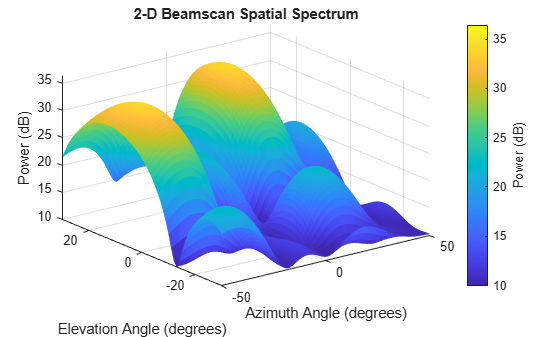# phased.BeamscanEstimator2D

2-D beamscan spatial spectrum estimator

## Description

The `phased.BeamscanEstimator2D` System object™ calculates a beamscan 2-D spatial spectrum estimate for an arbitrary array (ULA). The object estimates the incoming signal spatial spectrum using a narrowband conventional beamformer.

To estimate the spatial spectrum:

1. Create the `phased.BeamscanEstimator2D` object and set its properties.

2. Call the object with arguments, as if it were a function.

## Creation

### Syntax

``estimator = phased.BeamscanEstimator2D``
``estimator = phased.BeamscanEstimator2D(Name,Value)``

### Description

````estimator = phased.BeamscanEstimator2D` creates a beamscan 2-D spatial spectrum estimator System object.```
````estimator = phased.BeamscanEstimator2D(Name,Value)` creates an object, `estimator`, with each specified property `Name` set to the specified `Value`. You can specify additional name-value pair arguments in any order as (`Name1`,`Value1`,...,`NameN`,`ValueN`).```

## Properties

expand all

Unless otherwise indicated, properties are nontunable, which means you cannot change their values after calling the object. Objects lock when you call them, and the `release` function unlocks them.

If a property is tunable, you can change its value at any time.

Sensor array, specified as a Phased Array System Toolbox array System object.

Example: `phased.URA`

Signal propagation speed, specified as a real-valued positive scalar. Units are in meters per second. The default propagation speed is the value returned by `physconst('LightSpeed')`.

Example: `3e8`

Data Types: `single` | `double`

Operating frequency, specified as a positive scalar. Units are in Hz.

Example: `1e9`

Data Types: `single` | `double`

The number of bits used to quantize the phase shift component of beamformer or steering vector weights, specified as a non-negative integer. A value of zero indicates that no quantization is performed.

Example: `5`

Data Types: `single` | `double`

Enable forward-backward averaging, specified as `false` or `true`. Set this property to `true` to use forward-backward averaging to estimate the covariance matrix for sensor arrays with a conjugate symmetric array manifold.

Data Types: `logical`

Azimuth scan angles, specified as a or real-valued row vector. Angle units are in degrees. The angle values must lie between –180° and 180°, inclusive, and be in ascending order.

Example: `[-30:20]`

Data Types: `single` | `double`

Elevation scan angles, specified as a real-valued row vector. Angle units are in degrees. The angle values must lie between –90° and 90°, inclusive, and be in ascending order.

Example: `[-70:75]`

Data Types: `single` | `double`

Option to enable directions-of-arrival (DOA) output, specified as `false` or `true`. To obtain the DOA of signals, set this property to `true`. The DOAs are returned in the second output argument when the object is executed.

Data Types: `logical`

Number of arriving signals for DOA estimation, specified as a positive integer.

Example: `3`

#### Dependencies

To enable this property, set the `DOAOutputPort` property to `true`.

Data Types: `single` | `double`

## Usage

### Syntax

``Y = estimator(X)``
``[Y,ANG] = estimator(X)``

### Description

````Y = estimator(X)` estimates the spatial spectrum from data `X`.The size of the first dimension of the input matrix can vary to simulate a changing signal length. A size change can occur, for example, in the case of a pulse waveform with variable pulse repetition frequency.```

example

````[Y,ANG] = estimator(X)` returns the directions of arrival, `ANG`, of the signals. To enable this syntax, set the `DOAOutputPort` property to `true`. `ANG` is a 2-by-N matrix of the estimated azimuths and elevations of the signal direction. N is specified by the `NumSignals` property. If the object cannot identify a signal direction, it will return `NaN`.```

Note

The object performs an initialization the first time the object is executed. This initialization locks nontunable properties and input specifications, such as dimensions, complexity, and data type of the input data. If you change a nontunable property or an input specification, the System object issues an error. To change nontunable properties or inputs, you must first call the `release` method to unlock the object.

### Input Arguments

expand all

Array data, specified as a complex-valued matrix. Columns of the data matrix correspond to channels.

Data Types: `single` | `double`
Complex Number Support: Yes

### Output Arguments

expand all

Magnitude of the estimated spatial spectrum, returned as a positive, real-valued, K-by-L matrix.

Data Types: `single` | `double`

Estimated direction angles of signal arrivals, returned as a real-valued 2-by-K matrix. Each column has the form `[azimuth;elevation]`. The `NaN` value in any matrix element indicates that an estimate could not be found. Units are in degrees.

Data Types: `single` | `double`

## Object Functions

To use an object function, specify the System object as the first input argument. For example, to release system resources of a System object named `obj`, use this syntax:

`release(obj)`

expand all

 `plotSpectrum` Plot spatial spectrum
 `step` Run System object algorithm `release` Release resources and allow changes to System object property values and input characteristics `reset` Reset internal states of System object

## Examples

collapse all

Estimate the DOAs of two signals received by a 50-element URA with a rectangular lattice. The antenna operating frequency is 150 MHz. The actual direction of the first signal is -37° in azimuth and 0° in elevation. The direction of the second signal is 17° in azimuth and 20° in elevation.

```antenna = phased.IsotropicAntennaElement('FrequencyRange',[100e6 300e6]); array = phased.URA('Element',antenna,'Size',[5 10],'ElementSpacing',[1 0.6]); fc = 150e6; lambda = physconst('LightSpeed')/fc; ang1 = [-37.5; 10.2]; ang2 = [17.4; 20.6]; x = sensorsig(getElementPosition(array)/lambda,8000,[ang1 ang2],0.2); estimator = phased.BeamscanEstimator2D('SensorArray',array,'OperatingFrequency',fc, ... 'DOAOutputPort',true,'NumSignals',2,'AzimuthScanAngles',-50:50,'ElevationScanAngles',-30:30); [~,doas] = estimator(x); disp(doas)```
``` 17 -37 20 10 ```

Because the values for the Azimuth`ScanAngles` and ElevationScanAngles properties have a granularity of ${1}^{\circ }$, the DOA estimates are not accurate. Improve the accuracy by choosing a finer grid

```estimator2 = phased.BeamscanEstimator2D('SensorArray',array,'OperatingFrequency',fc, ... 'DOAOutputPort',true,'NumSignals',2,'AzimuthScanAngles',-50:0.05:50,'ElevationScanAngles',-30:0.05:30); [~,doas] = estimator2(x); disp(doas)```
``` 17.3000 -37.4000 20.5000 10.3000 ```

Plot the beamscan spatial spectrum

`plotSpectrum(estimator)`## Algorithms

expand all

 Van Trees, H. Optimum Array Processing. New York: Wiley-Interscience, 2002.